# Olympiad Test: Fractions and Decimals

Test Description

## 20 Questions MCQ Test Advance Learner Course: Mathematics (Maths) Class 6 | Olympiad Test: Fractions and Decimals

Olympiad Test: Fractions and Decimals for Class 7 2023 is part of Advance Learner Course: Mathematics (Maths) Class 6 preparation. The Olympiad Test: Fractions and Decimals questions and answers have been prepared according to the Class 7 exam syllabus.The Olympiad Test: Fractions and Decimals MCQs are made for Class 7 2023 Exam. Find important definitions, questions, notes, meanings, examples, exercises, MCQs and online tests for Olympiad Test: Fractions and Decimals below.
 1 Crore+ students have signed up on EduRev. Have you?
Olympiad Test: Fractions and Decimals - Question 1

### A __________ is a fraction that represents a part of a whole.

Detailed Solution for Olympiad Test: Fractions and Decimals - Question 1

A proper fraction is a number representing a part of a whole. The whole may be a single object or a group of objects.

Example: Consider the fraction 7/12. This fraction is read as ”seven-twelfth” which means that 7 parts out of 12 equal parts in which the whole is divided.
In the fraction 7/12, 7 is called the numerator and 12 is called the denominator.

Olympiad Test: Fractions and Decimals - Question 2

### Three times the first of three consecutive odd integers is 3 more than twice the third. The third integer is:

Detailed Solution for Olympiad Test: Fractions and Decimals - Question 2

Let the three integers be: xx + 2 and x + 4.
Then, 3x = 2(x + 4) + 3

► x = 11.

Third integer = x + 4 = 15.

Olympiad Test: Fractions and Decimals - Question 3

### The product of two numbers is 2.0016. If one of them is 0.72, find the other number.

Detailed Solution for Olympiad Test: Fractions and Decimals - Question 3

Product of two numbers = 2.0016
One number = 0.72
Other number = (2.0016 ÷ 0.72)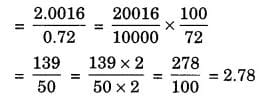Hence, the required number = 2.78

Olympiad Test: Fractions and Decimals - Question 4

If 2994 ÷ 14.5 = 172, then 29.94 ÷ 1.45 = ?

Detailed Solution for Olympiad Test: Fractions and Decimals - Question 4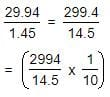[ Here, Substitute 172 in the place of 2994/14.5 ]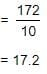Olympiad Test: Fractions and Decimals - Question 5

The price of commodity X increases by 40 paise every year, while the price of commodity Y increases by 15 paise every year. If in 2001, the price of commodity X was Rs. 4.20 and that of Y was Rs. 6.30, in which year commodity X will cost 40 paise more than the commodity Y ?

Detailed Solution for Olympiad Test: Fractions and Decimals - Question 5

Suppose commodity X will cost 40 paise more than Y after Z  years. Then,

► (4.20 + 0.40Z) - (6.30 + 0.15Z) = 0.40
► 0.25Z = 0.40 + 2.10
► Z = (2.50 / 0.25) = 10

∴ X will cost 40 paise more than Y 10 years after 2001 i.e in 2011.

Olympiad Test: Fractions and Decimals - Question 6

A fraction acts as an operator ________.

Detailed Solution for Olympiad Test: Fractions and Decimals - Question 6

Fraction as an operator ‘of ’.
Fraction acts as an operator of  'of' represents multiplication.

Example: 1/3 of 90 = 1/3 × 90 = 30.

Olympiad Test: Fractions and Decimals - Question 7

The product of two proper fractions is ________ each of the fractions that are multiplied.

Detailed Solution for Olympiad Test: Fractions and Decimals - Question 7

When two proper fractions are multiplied, the product is less than both the fractions. Or, we say the value of the product of two proper fractions is smaller than each of the two fractions.

Olympiad Test: Fractions and Decimals - Question 8

In a class of 40 students 1/5 of the total number of students like to study English, 2/5 of the total number like to study mathematics and the remaining students like to study Science.

Q. How many students like to study English?

Detailed Solution for Olympiad Test: Fractions and Decimals - Question 8

Number of students who like english = 1/5 of 40

= 1/5 ×40
= 8 students

Olympiad Test: Fractions and Decimals - Question 9

What will be the vulgar fraction of 0.75

Detailed Solution for Olympiad Test: Fractions and Decimals - Question 9

► 0.75 = (75 / 100) = 3/4

Olympiad Test: Fractions and Decimals - Question 10

Find the average of 4.2, 3.8 and 7.6.

Detailed Solution for Olympiad Test: Fractions and Decimals - Question 10

Average = Sum of numbers / Total quantity of numbers

= (4.2 + 3.8 + 7.6) / 3
= 15.6 / 3
= 5.2

Olympiad Test: Fractions and Decimals - Question 11

(2 / 7) × 3 =_______

Detailed Solution for Olympiad Test: Fractions and Decimals - Question 11

► (2 / 7) × 3 = 6 / 7

Olympiad Test: Fractions and Decimals - Question 12

There is a tree between houses of A and B. If the tree leans on A’s House, the tree top rests on his window which is 12 m from ground. If the tree leans on B’s House, the tree top rests on his window which is 9 m from ground. If the height of the tree is 15 m, what is distance between A’s and B’s house?

Detailed Solution for Olympiad Test: Fractions and Decimals - Question 12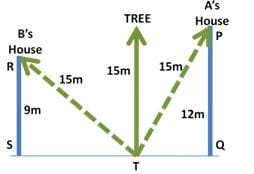In ΔSTR, By Pythagoras theorem:
RT2 = ST2 + RS2

► ST2 = 152 - 92 = 144
► ST = 12m

In ΔTQP, By Pythagoras theorem:
PT2 = TQ2 + PQ2

► TQ2 = 152 - 122 = 81
► TQ = 9m

Distance between houses = SQ = ST + TQ = 12 + 9 = 21m

Olympiad Test: Fractions and Decimals - Question 13

What decimal of an hour is a second ?

Detailed Solution for Olympiad Test: Fractions and Decimals - Question 13

Required decimal =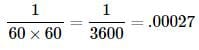Olympiad Test: Fractions and Decimals - Question 14

(2 / 5) ÷  7 = ______.

Detailed Solution for Olympiad Test: Fractions and Decimals - Question 14

► (2 / 5) x (1 / 7) = (2 / 35)

Olympiad Test: Fractions and Decimals - Question 15

0.02 × 0.03 = ______

Detailed Solution for Olympiad Test: Fractions and Decimals - Question 15

0.02 × 0.03 = 0.0006

Olympiad Test: Fractions and Decimals - Question 16

In proper fraction, the numerator is ________ the denominator.

Detailed Solution for Olympiad Test: Fractions and Decimals - Question 16

In a proper fraction, the numerator is always less than the denominator.
Examples of proper fractions include:
1/2, 9/13 and 1/1001.

Olympiad Test: Fractions and Decimals - Question 17

Reciprocal of 7 / 2 is _____

Detailed Solution for Olympiad Test: Fractions and Decimals - Question 17

A reciprocal of a fraction a / b is created by changing places of numerator and denominator (it can only be done if the numerator of the original fraction a is different from zero), so it is b / a.

So, in the given example the reciprocal of 7/2 is 2 / 7.

Olympiad Test: Fractions and Decimals - Question 18

In a class 3/4th of the students do not know either English or Hindi. But 1/6th of the students know English. How much students know both English and Hindi if students who know Hindi are 1/8th of total students in the class?

Detailed Solution for Olympiad Test: Fractions and Decimals - Question 18

3 / 4th do not know English or Hindi.
So, 1 / 4th know English or Hindi or both.
1/6th know English and 1/8th know Hindi .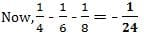Olympiad Test: Fractions and Decimals - Question 19

(1/3) of 3 is ____

Detailed Solution for Olympiad Test: Fractions and Decimals - Question 19

(⅓) of 3 means 1/3 * 3 = 1.
∵ 3 in numerator and denominator cancels out.

Olympiad Test: Fractions and Decimals - Question 20

Multiply 2.05 and 1.3.

Detailed Solution for Olympiad Test: Fractions and Decimals - Question 20

When you multiply 2.05 by 1.3 you get 2.665 , because there are three decimal places.

## Advance Learner Course: Mathematics (Maths) Class 6

33 videos|21 docs|9 tests
 Use Code STAYHOME200 and get INR 200 additional OFF Use Coupon Code